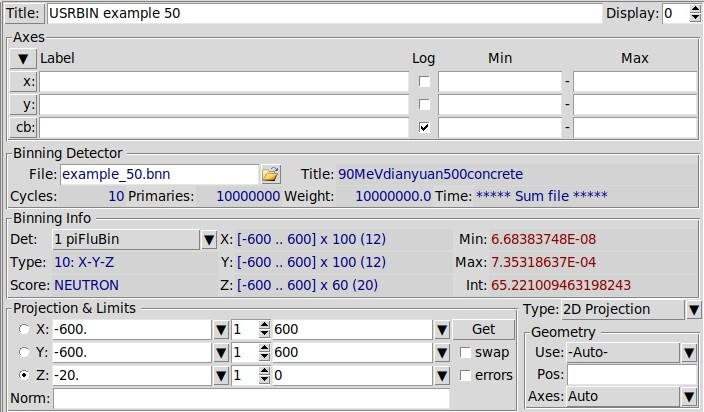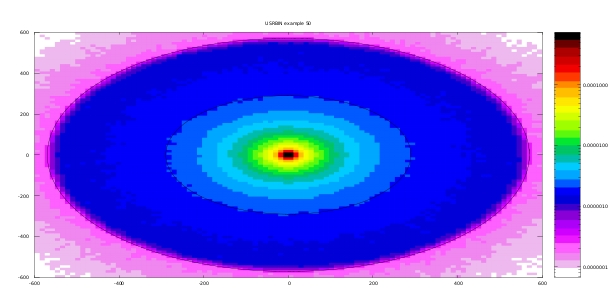# How to calculate neutron dose rate?

Dear experts,
I want to know how to calculate neutron dose rate in USRBIN ?
Which one should I choose in WHAT(2) and what is the unit of the result ?

Dear Junjie,

Could you give a bit more details on what exactly you want to calculate? Through USRBIN scoring you can calculate the neutron fluence by setting WHAT(1) >= 10 and “NEUTRON” as WHAT(2), see the FLUKA manual on USRBIN. In each bin the results will be expressed as amount of particles per cm^2 per unit primary weight.

In case you would like to score dose (in USRBIN card set WHAT(2) = DOSE) or dose equivalent (WHAT(2) = DOSE-EQ) and you want to single out the contribution from neutrons only you can associate an AUXSCORE card to the USRBIN (named let’s say “bin”) with WHAT(1) = USRBIN, WHAT(2) = NEUTRON, WHAT(4) = bin.

Note that in these cases FLUKA will not yield rates as an output, if you would like to express the results per unit time you will need to multiply the results by an appropriate factor such as e.g. irradiation intensity. Does this answer your questions?

Best regards,

Andreas

My problem is a radiation shielding problem. I need to calculate the influence of neutrons at different positions produced by proton hitting targets , so I want to calculate neutron dose rates at different positions by FLUKA. I want to know which “WHAT(2)”（NEUTRON, DOSE or DOSE-EQ) should I choose in USRBIN and how to deal with the results of USRBIN to get the dose rate？

Hi Junjie,

Depending on what exactly you want to know at the different positions you mention there are several options to set for WHAT(2):

• NEUTRON will simply calculate the neutron fluence and results will be expressed in each bin as neutrons/cm^2 per unit primary.

• DOSE will calculate the total absorbed dose which will be expressed in GeV/g per unit primary weight. To obtain the dose in Gray [Gy] you need to multiply the result in GeV/g by 1.602176462E-7. To single out only the contribution due to neutrons only, include the AUXSCORE card as I explained in the previous post.

• DOSE-EQ will calculate the dose equivalent which means the fluence is multiplied with material and energy dependent conversion coefficients to yield an effective dose expressed as pSv (pico-Sievert) per unit primary weight. Again for this option you need to include the AUXSCORE card to select only neutrons.

All results will be expressed per unit primary weight, i.e. per single primary particle that you simulate using FLUKA, which in your case is a proton. Therefore, to express the results as a rate you need to multiply by the proton intensity, the amount of protons per unit time hitting your target. Does this help?

Best regards,

Andreas

Thank you very much for your answer. I have roughly understood the result of each option.
I put the USRBIN card and the results in the picture. here are my questions:

1. Are the results obtained by these two cards neutron fluence?
2. Is the result the average neutron fluence in Z direction?
3. If I change “WHAT(2)” in USRBIN to DOSE-EQ, can I get the neutron dose equivalent?
4. You said in the above answer that the dose equivalent rate can be obtained by multiplying the dose equivalent by proton intensity. What is the standard unit of proton intensity?
I’m sorry to trouble you again, and I sincerely wait for your reply.Hi Junjie,

1. In the screenshot that you show, the first “piFluBin” USRBIN card scores the neutron fluence. The second card which is the AUXSCORE you associate with the USRBIN “piFluBin” to select only neutrons is not necessary: this is already done by setting WHAT(2) in the USRBIN card to “NEUTRON”. As a side remark: you specified the AUXSCORE SDUM to “EWT74” which refers to a set of dose conversion coefficients. Hence this is only applicable when scoring dose equivalent (by setting “DOSE-EQ” as WHAT(2) in the USRBIN) and you wish to change the standard dose conversion coefficient set.

2. A fluence simply represents number of particles per unit surface and is independent of any direction, in FLUKA the results are expressed per [cm^2]. In each bin of your scoring mesh, FLUKA actually estimates the fluence as a density of particle track lengths: this makes sense since the length of a particle track is expressed per [cm] and density as [cm^3]. Putting this together we have for track-length density [cm/cm^3 = cm^2]. The track length itself is calculated as the distance the particle travels through a surface of infinitesimal thickness, you can find a more detailed explanation here.

3. Indeed if you change WHAT(2) to “DOSE-EQ” you get the dose equivalent but you need to include the AUXSCORE card to select only neutrons, the card you show in the screenshot is correct but note that with the SDUM = “EWT74” you change the conversion coefficient set from the default “AMB74” set to the “EWT74” set.

4. Depending on how you want to express your results you can define the proton intensity as you want. For example if you express the intensity as number of protons per second [s^-1], also the results for dose or dose-equivalent will be expressed per second. However you can choose to show results per hour, per day, … as well.